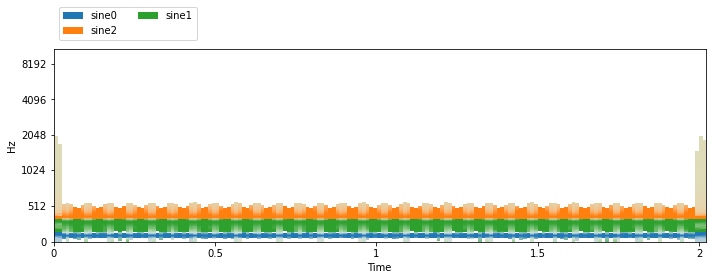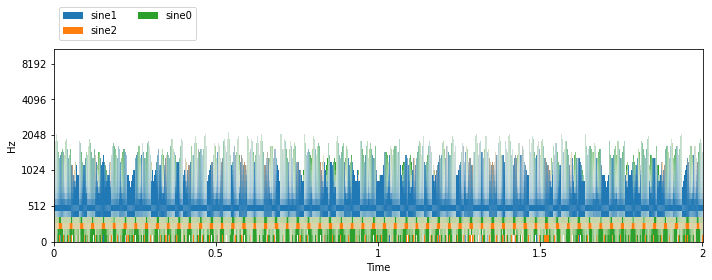# Handling data in nussl¶

nussl comes with a bunch of different useful dataset hooks, along with a handy base class for datasets. Let’s examine what the base class looks like first.

## BaseDataset¶

The BaseDataset is an abstract class that has a few useful functions for organizing your data. If you call it, directly, however, it will error out:

:

import nussl
import numpy as np
import matplotlib.pyplot as plt
import time

start_time = time.time()

folder = 'ignored'
base_dataset = nussl.datasets.BaseDataset(folder)

---------------------------------------------------------------------------
NotImplementedError                       Traceback (most recent call last)
<ipython-input-1-ee86740f50fb> in <module>
7
8 folder = 'ignored'
----> 9 base_dataset = nussl.datasets.BaseDataset(folder)

~/Dropbox/research/nussl_refactor/nussl/datasets/base_dataset.py in __init__(self, folder, transform, sample_rate, stft_params, num_channels, strict_sample_rate, cache_populated)
67                  num_channels=None, strict_sample_rate=True, cache_populated=False):
68         self.folder = folder
---> 69         self.items = self.get_items(self.folder)
70         self.transform = transform
71

~/Dropbox/research/nussl_refactor/nussl/datasets/base_dataset.py in get_items(self, folder)
131             list: list of items that should be processed
132         """
--> 133         raise NotImplementedError()
134
135     def __len__(self):

NotImplementedError:


For the dataset to work, two functions must be implemented:

1. self.get_items: A function that grabs all the items that you will need to process.

2. self.process_item: A function that processes a single item.

Let’s build a dataset that returns sums of sine wavs at random frequencies.

:

def make_sine_wave(freq, sample_rate, duration):
dt = 1 / sample_rate
x = np.arange(0.0, duration, dt)
x = np.sin(2 * np.pi * freq * x)
return x

class SineWaves(nussl.datasets.BaseDataset):
def get_items(self, folder):
# ignore folder and return a list
# 100 items in this dataset
items = list(range(100))
return items

def process_item(self, item):
# we're ignoring items and making
# sums of random sine waves
sources = {}
freqs = []
for i in range(3):
freq = np.random.randint(110, 1000)
freqs.append(freq)
_data = make_sine_wave(freq, self.sample_rate, 2)
# this is a helper function in BaseDataset for
# making an audio signal from data
sources[f'sine{i}'] = signal * 1/3

mix = sum(sources.values())

'frequencies': freqs
}

output = {
'mix': mix,
'sources': sources,
}
return output


The primary thing to note here is the format of what is output by the process_item function. It is a dictionary and must always be a dictionary. The dictionary contains three keys: mix, sources, and metadata. sources is similarly not a list but a dictionary. The sum of the values of sources adds up to mix.

Great, now let’s use this dataset.

:

folder = 'ignored'
sine_wave_dataset = SineWaves(folder, sample_rate=44100)

item = sine_wave_dataset
item

:

{'mix': <nussl.core.audio_signal.AudioSignal at 0x7fe6d592c690>,
'sources': {'sine0': <nussl.core.audio_signal.AudioSignal at 0x7fe6d592c2d0>,
'sine1': <nussl.core.audio_signal.AudioSignal at 0x7fe6d592c5d0>,
'sine2': <nussl.core.audio_signal.AudioSignal at 0x7fe6d592c310>},


We can see that getting an item from the dataset resulted in a dictionary containing AudioSignal objects! And the exact frequencies for each sine tone were saved in the metadata. Now, let’s listen and visualize:

:

def visualize_and_embed(sources, y_axis='mel'):
plt.figure(figsize=(10, 4))
plt.subplot(111)
sources, db_cutoff=-60, y_axis=y_axis)
plt.tight_layout()
plt.show()

nussl.play_utils.multitrack(sources, ext='.wav')

visualize_and_embed(item['sources'])The STFT parameters were inferred from the first time we used the dataset based on the audio signal’s sample rate and the defaults in nussl. To enforce a specific STFT parameter, we can do the following:

:

folder = 'ignored'
stft_params = nussl.STFTParams(window_length=256, hop_length=64)
sine_wave_dataset = SineWaves(folder, sample_rate=44100, stft_params=stft_params)

item = sine_wave_dataset
visualize_and_embed(item['sources'])
print('STFT shape:', item['mix'].stft().shape)